# MSBSHSE Solutions For Class 9 Maths Part 2 Chapter 9 Surface Area and Volume

MSBSHSE Solutions For Class 9 Maths Part 2 Chapter 9 Surface Area and Volume gives a clear understanding of the concept of Surface Area and Volume. These solutions are prepared by our subject specialists. This chapter deals with the surface area of the cube, cuboid, cylinder and cone. The volume of different three dimensional figures are also explained in this chapter. Students can easily download MSBSHSE Solutions For Class 9 Maths Part 2 Chapter 9 Surface Area and Volume from our website in PDF format.

## Download the PDF of Maharashtra Solutions For Class 9 Maths Part 2 Chapter 9 Surface Area and Volume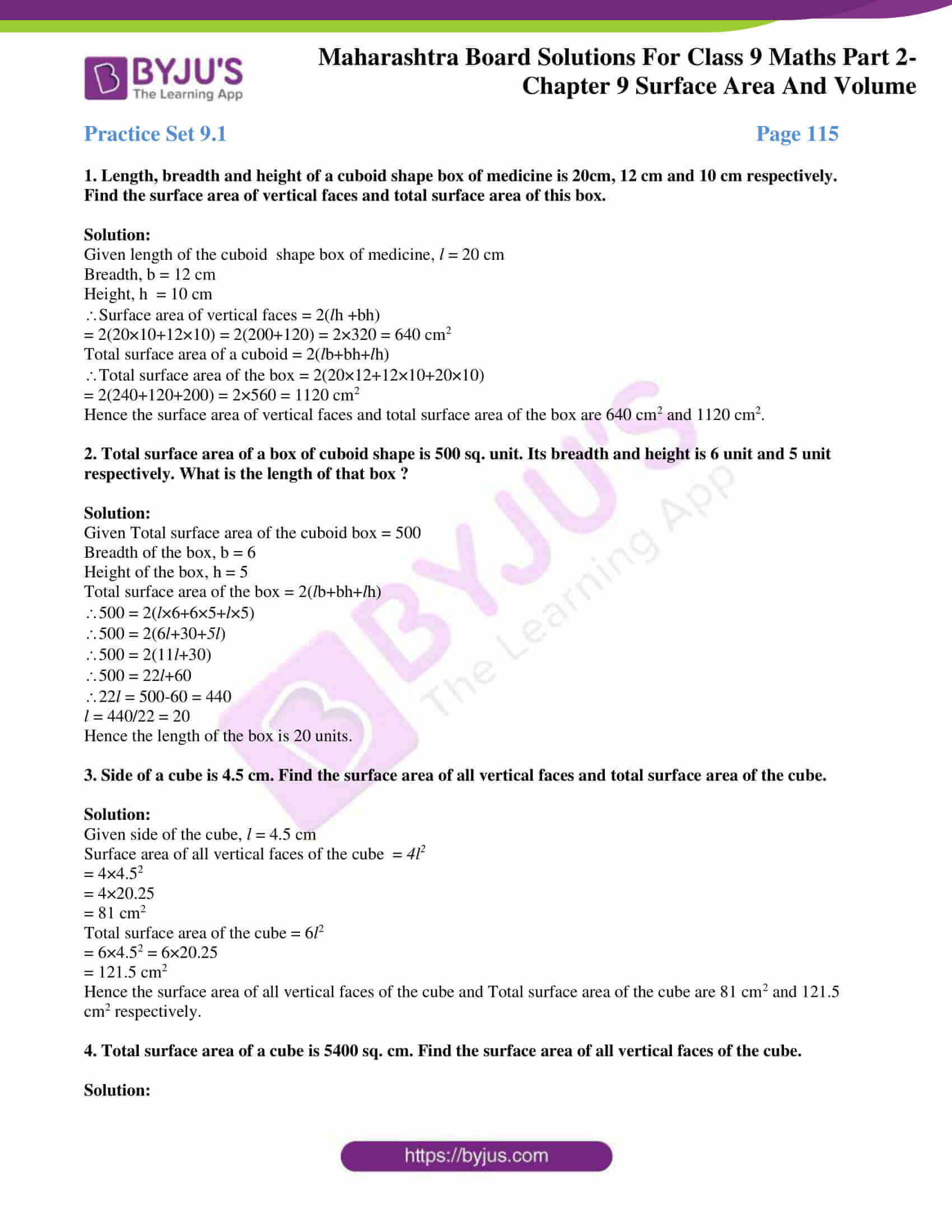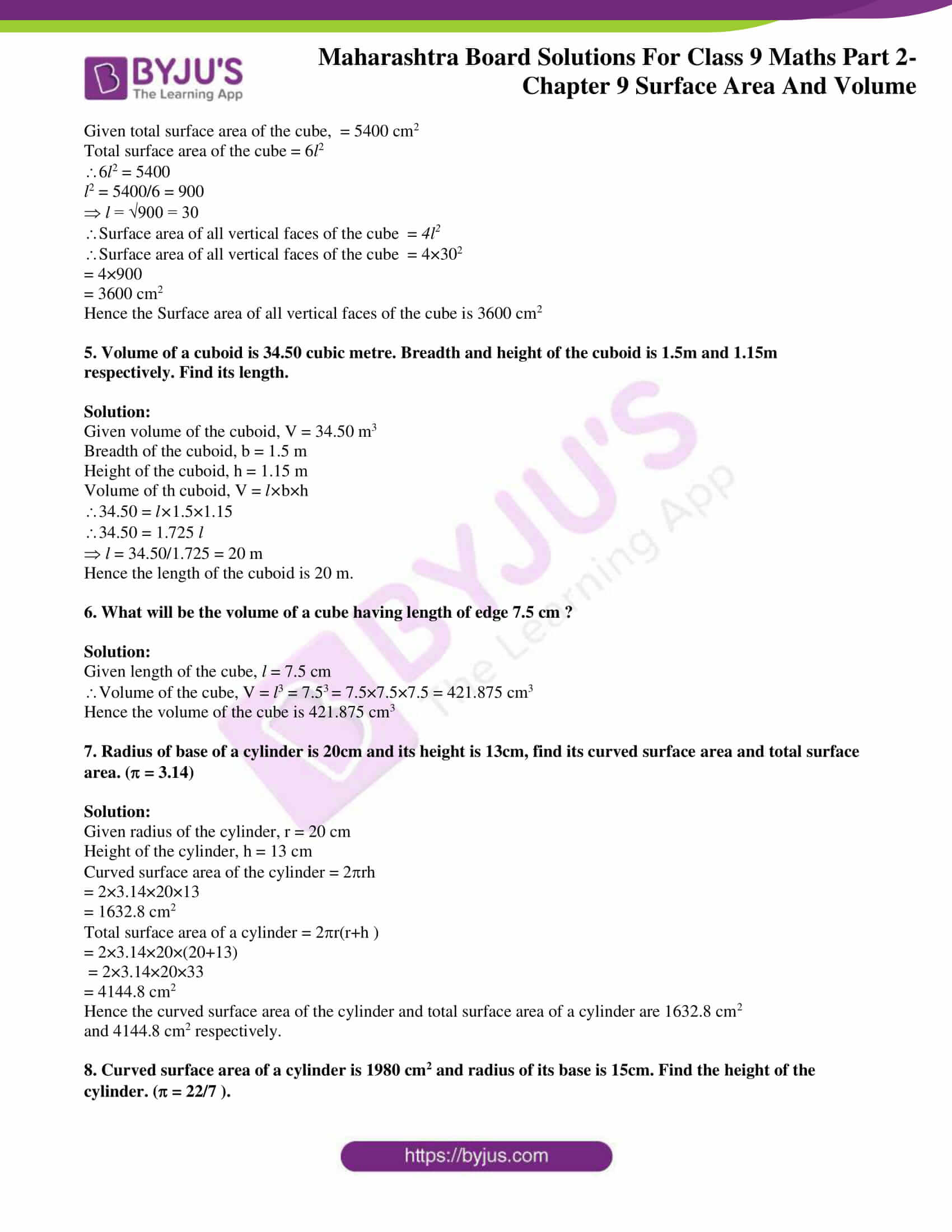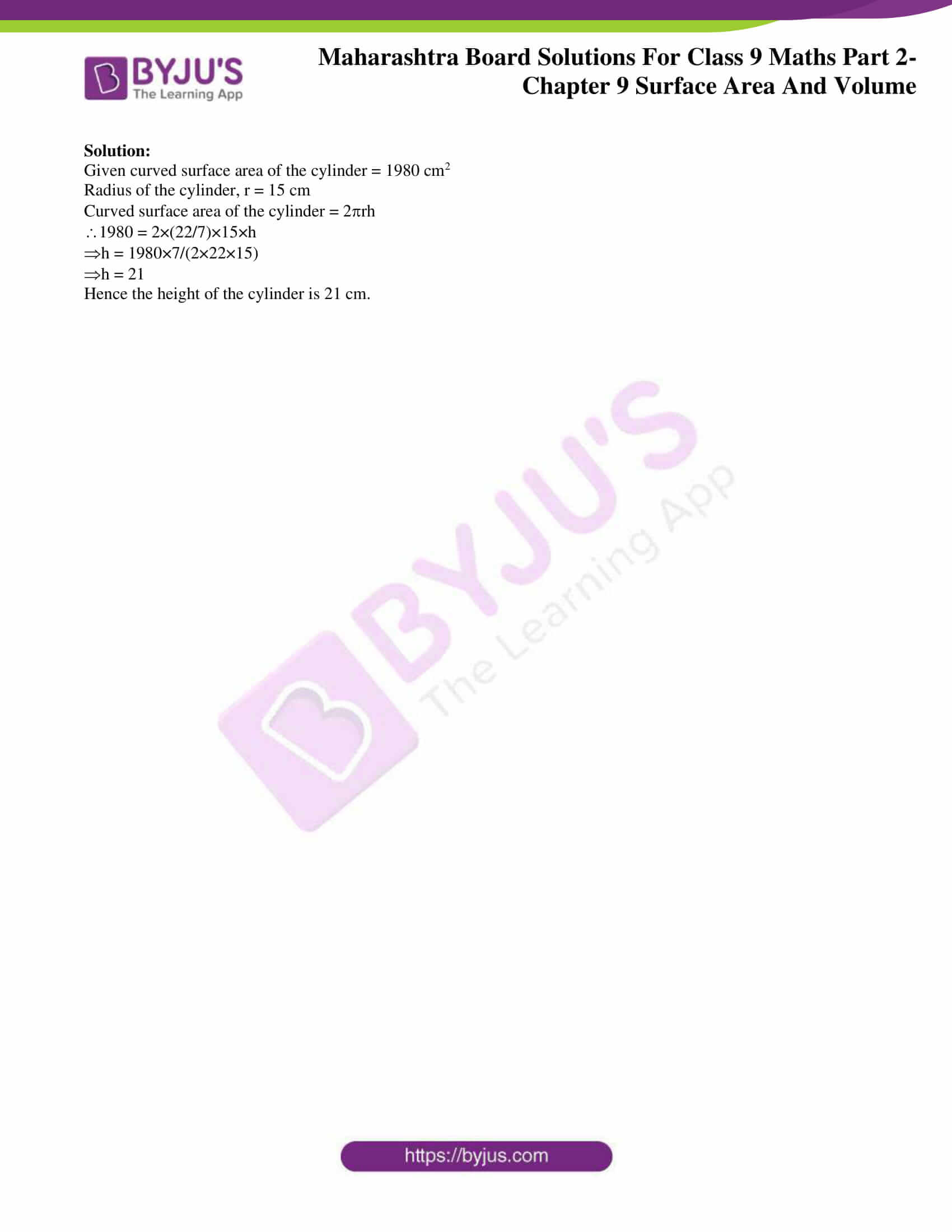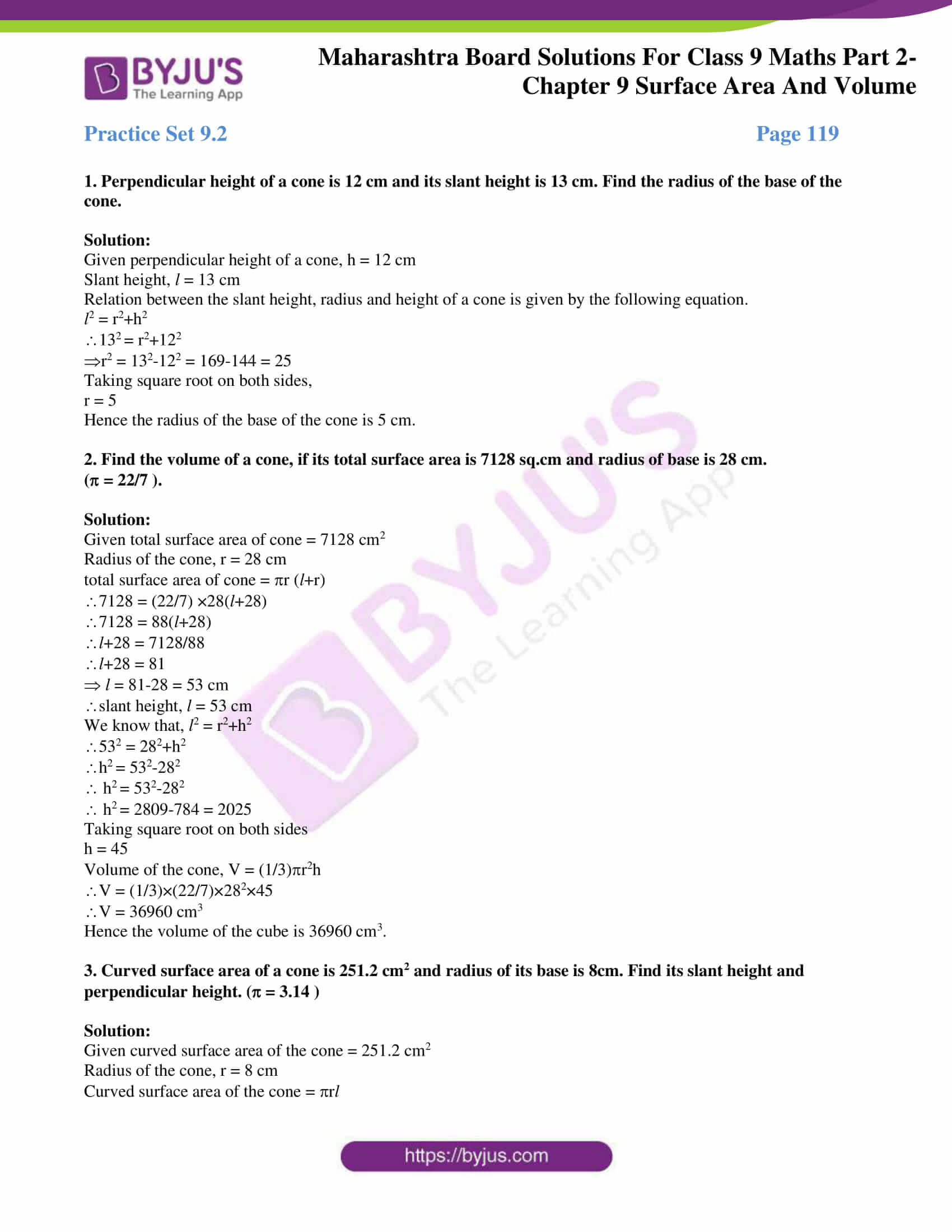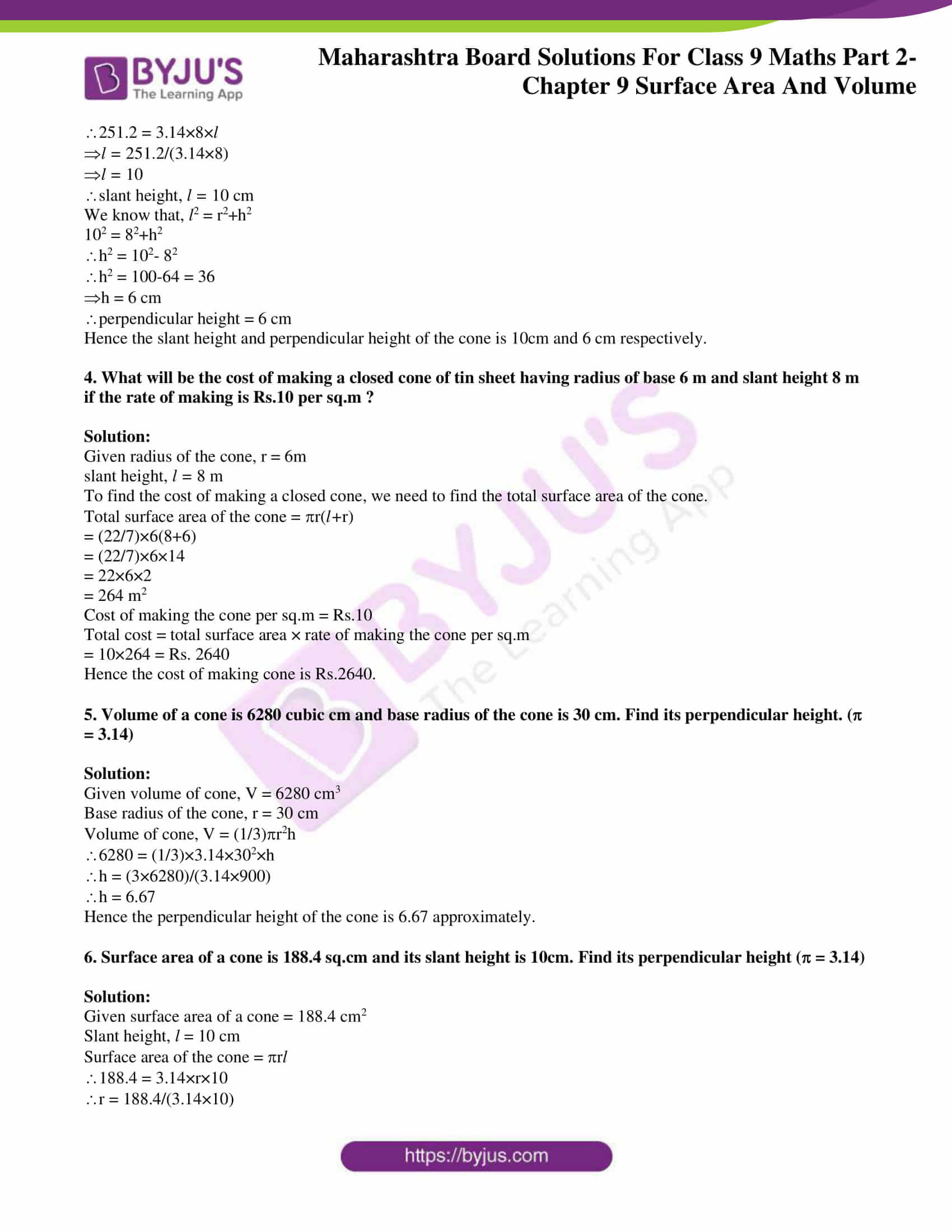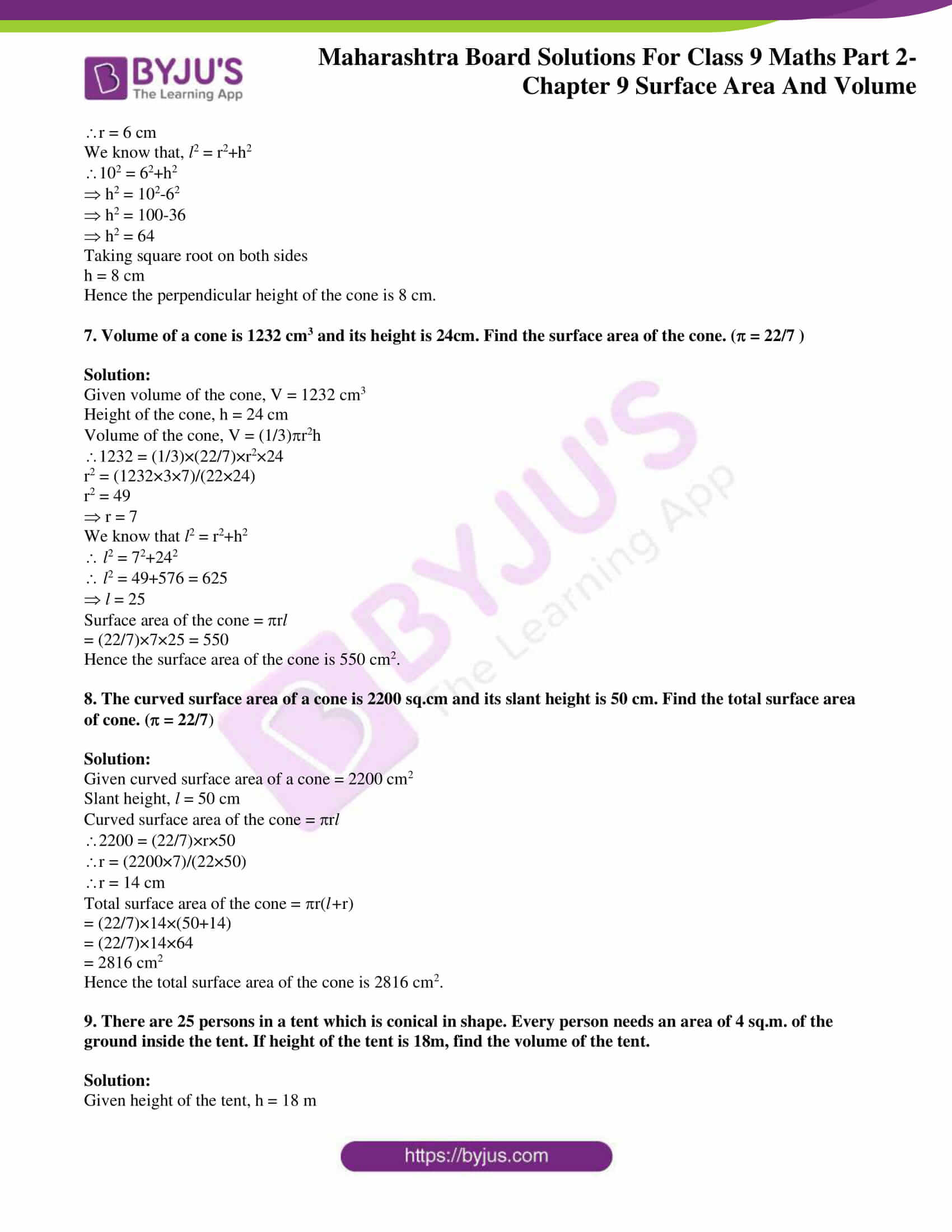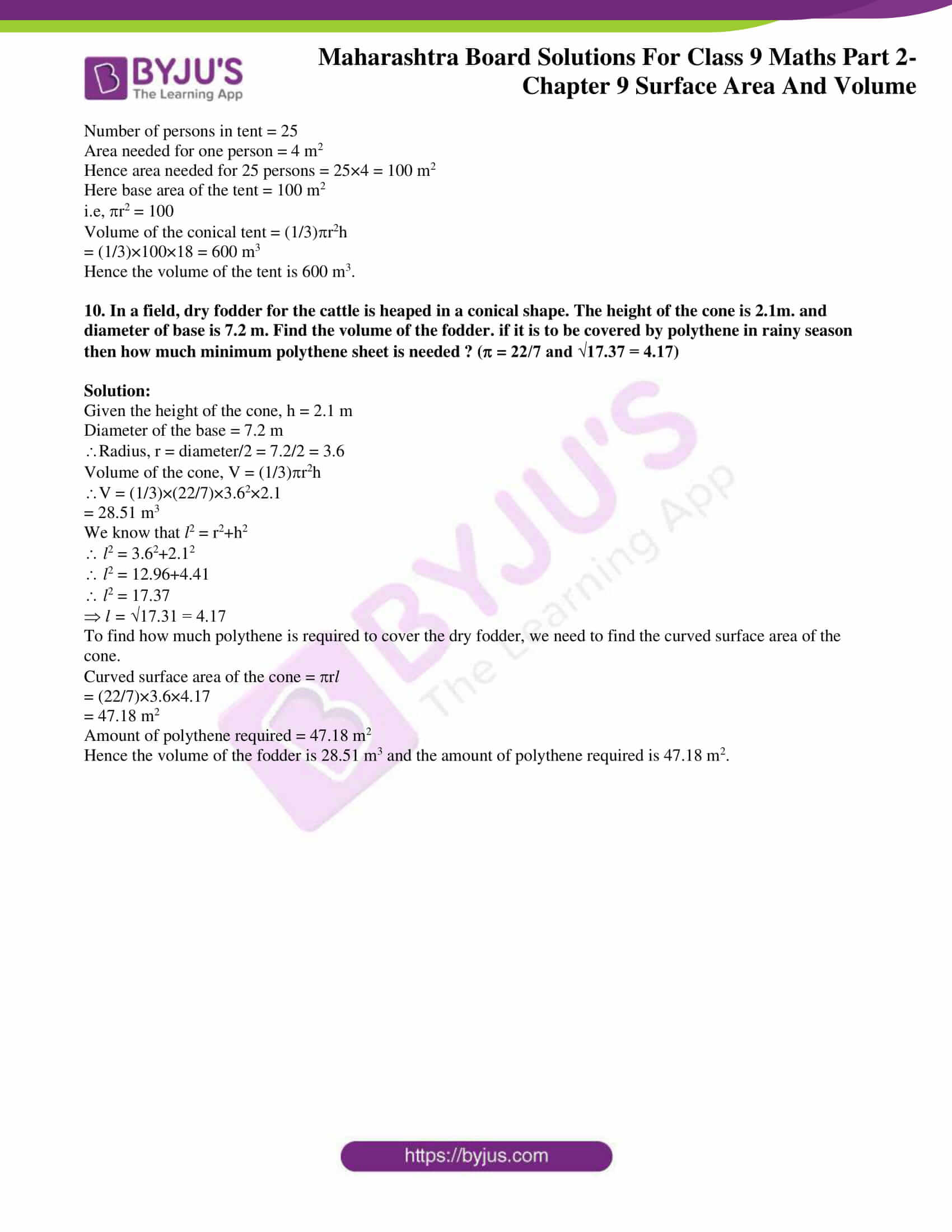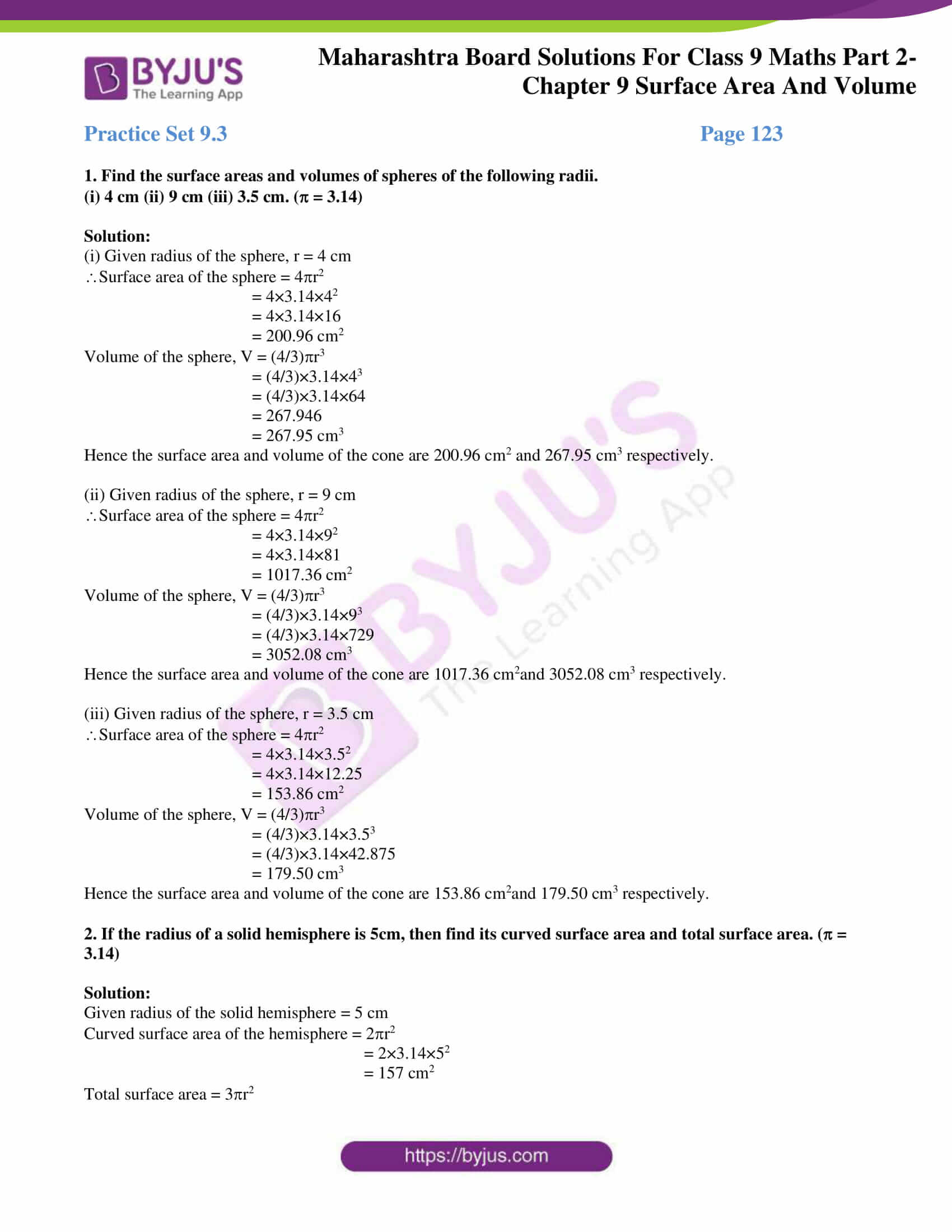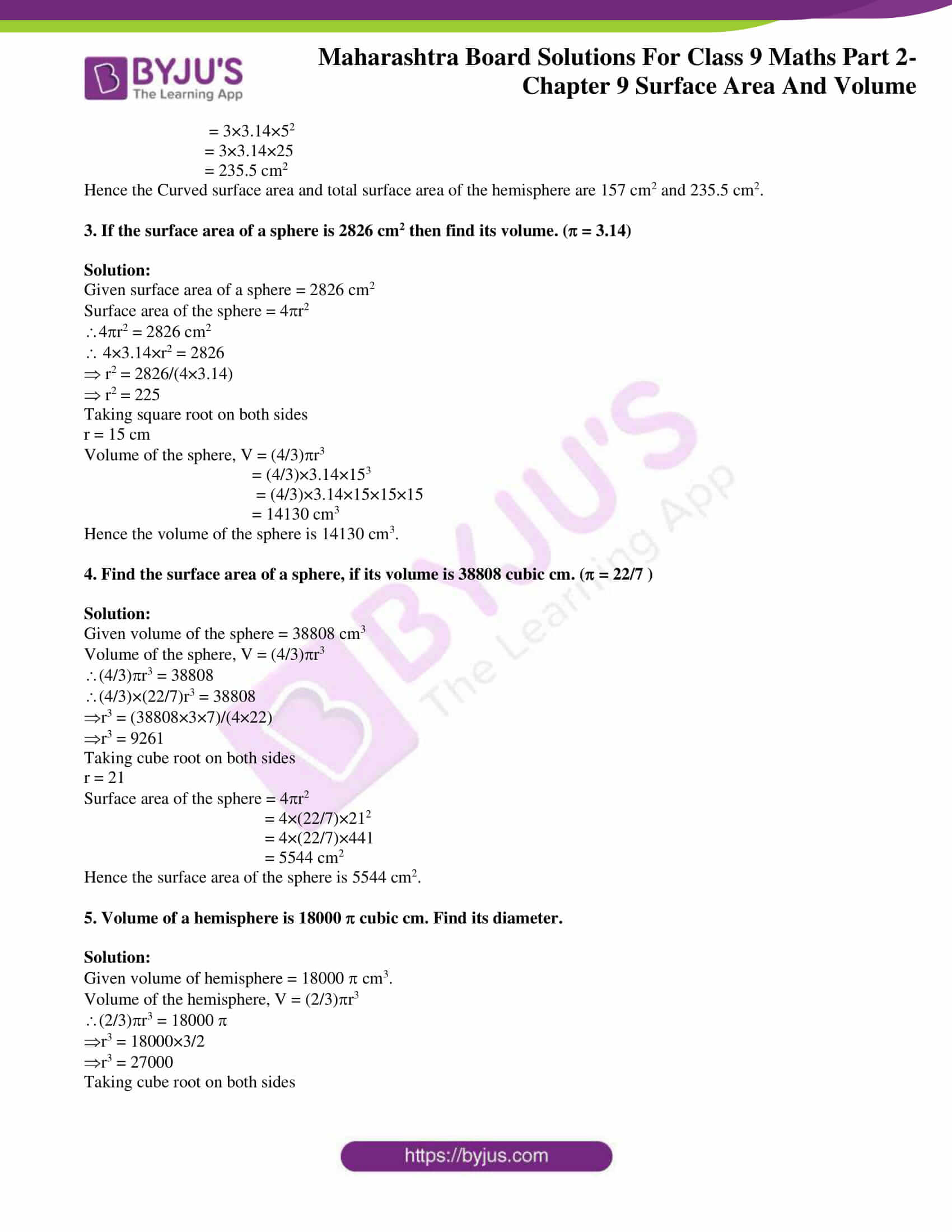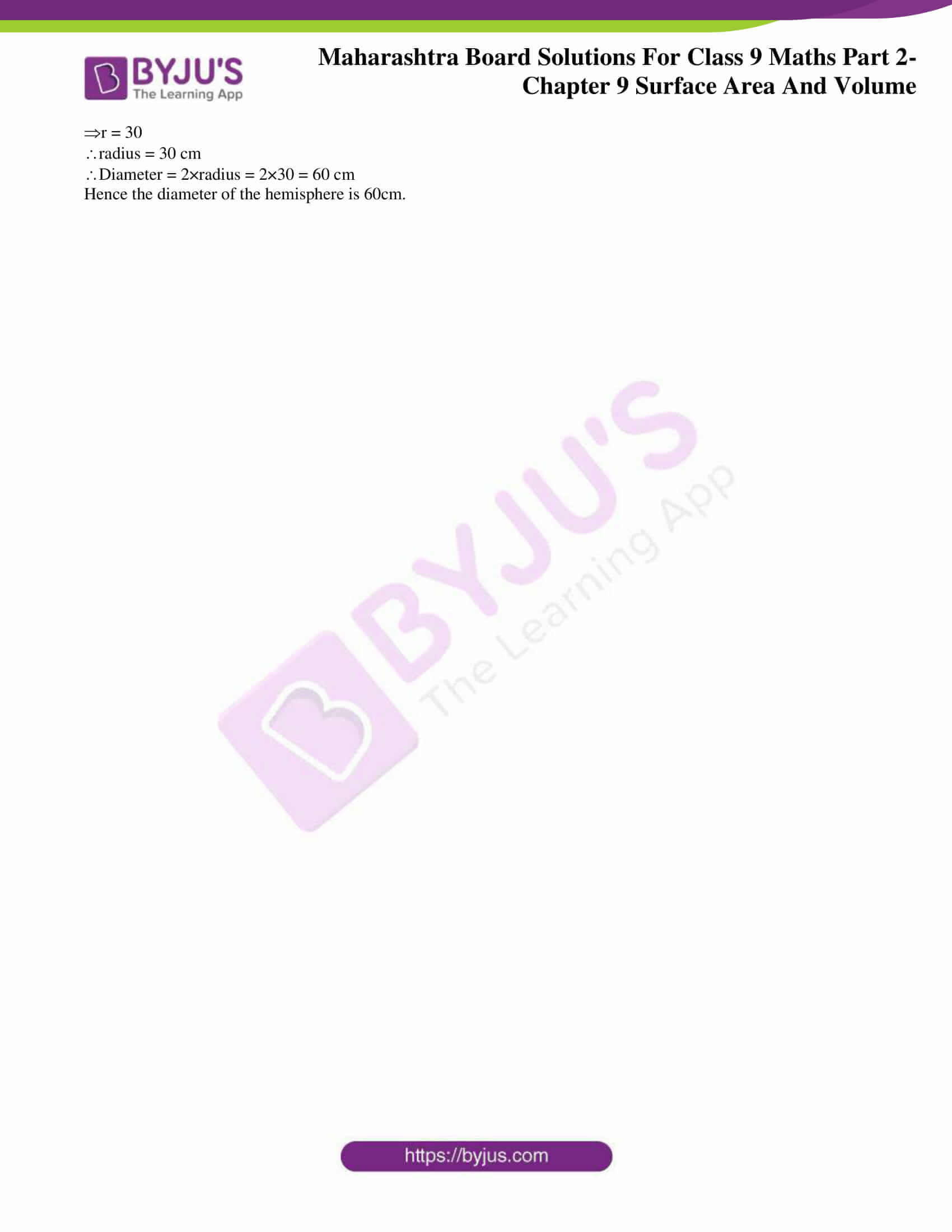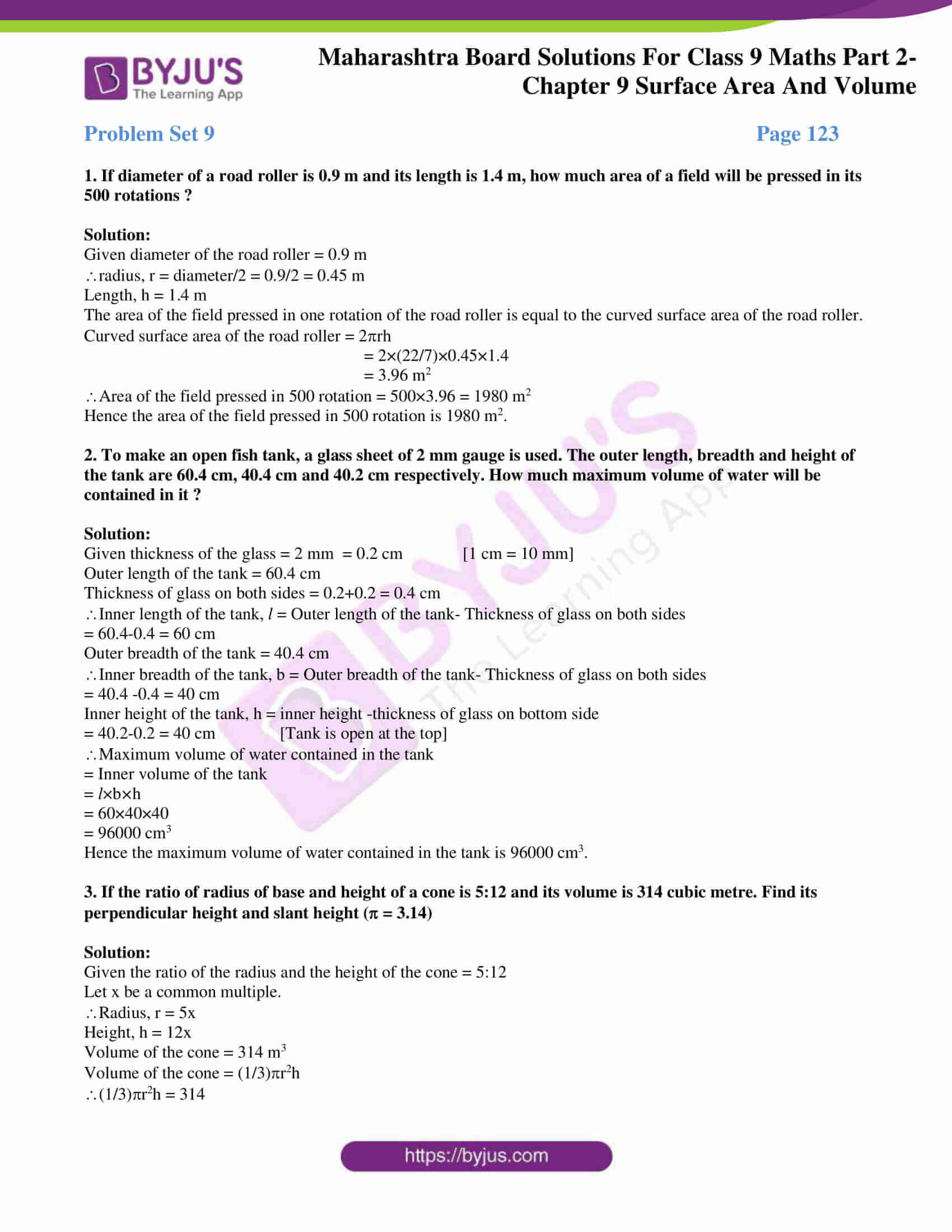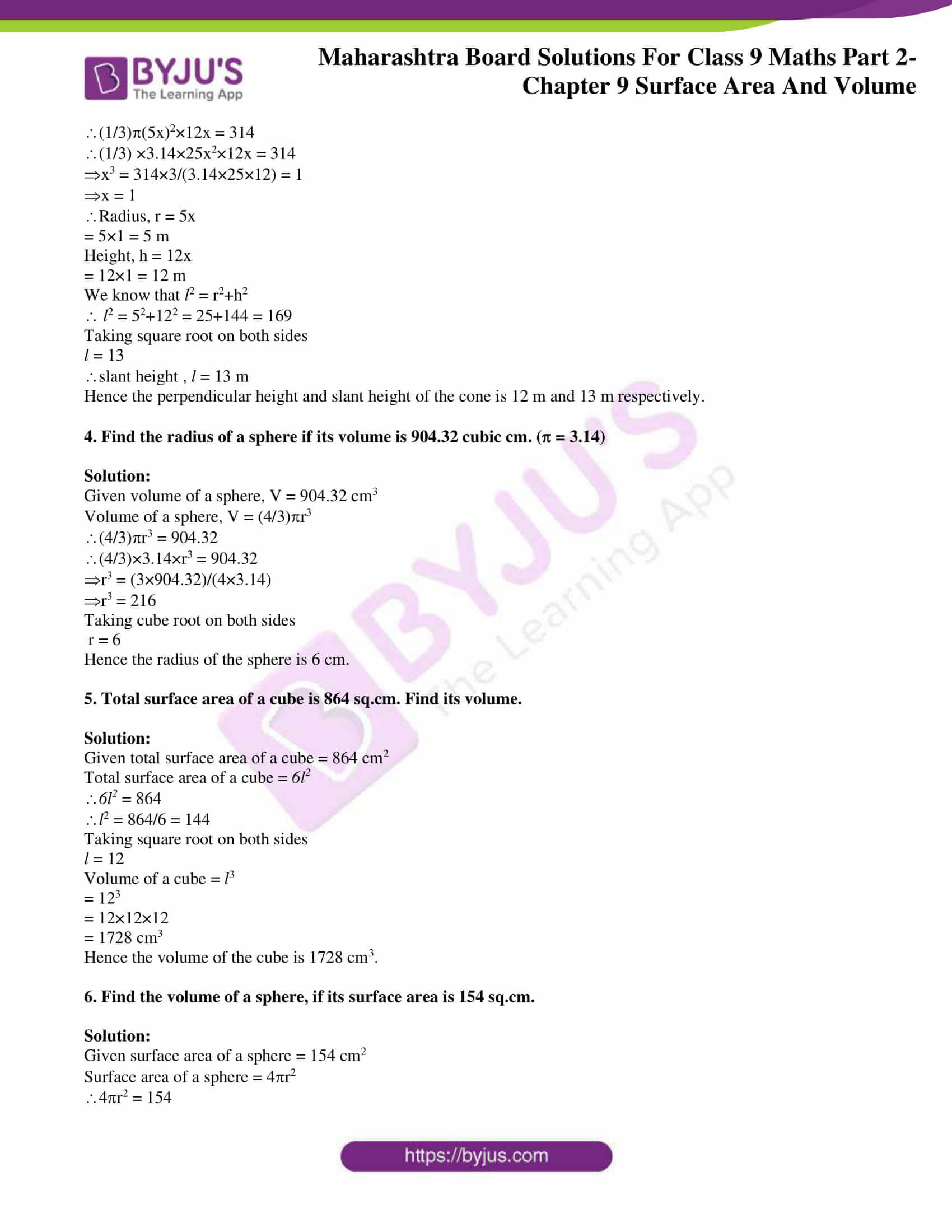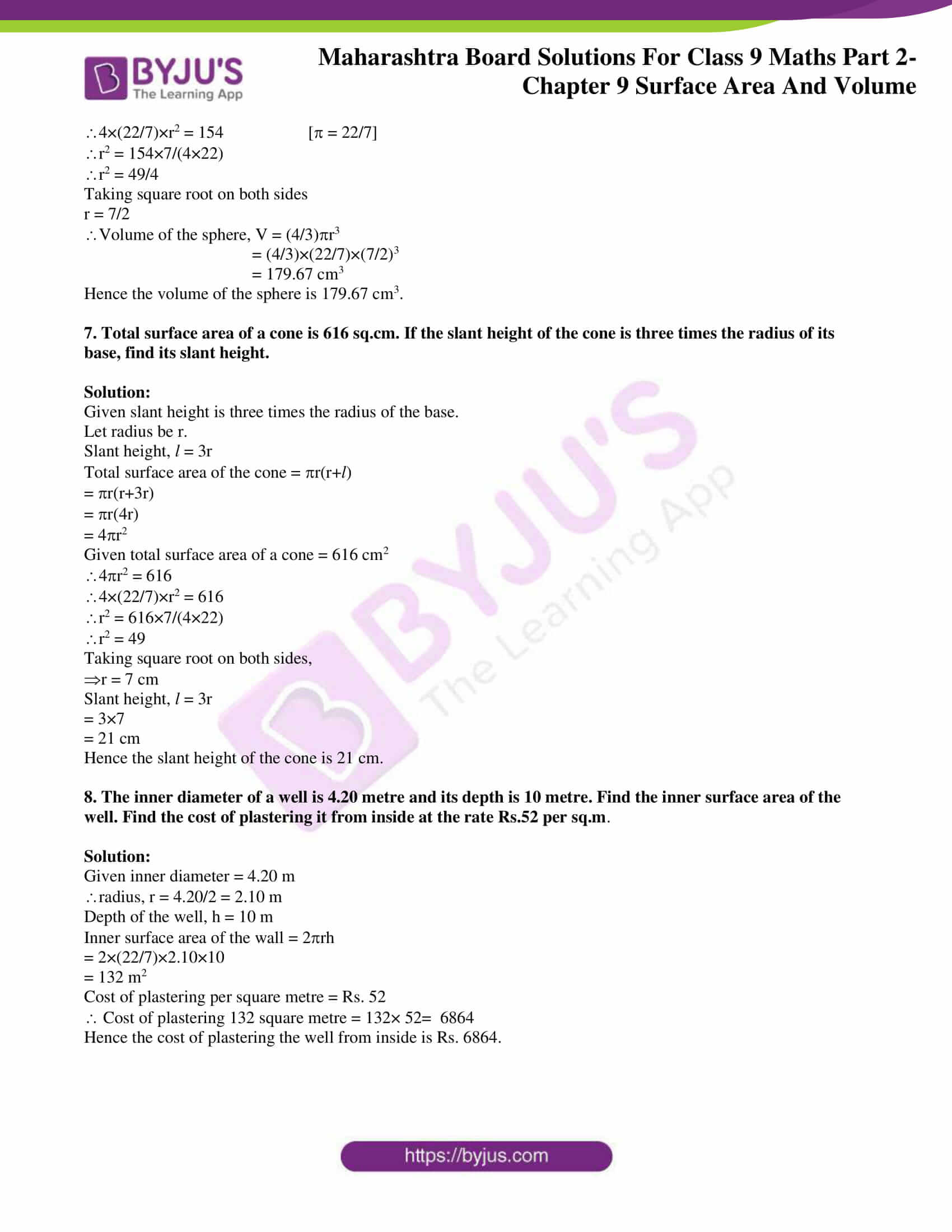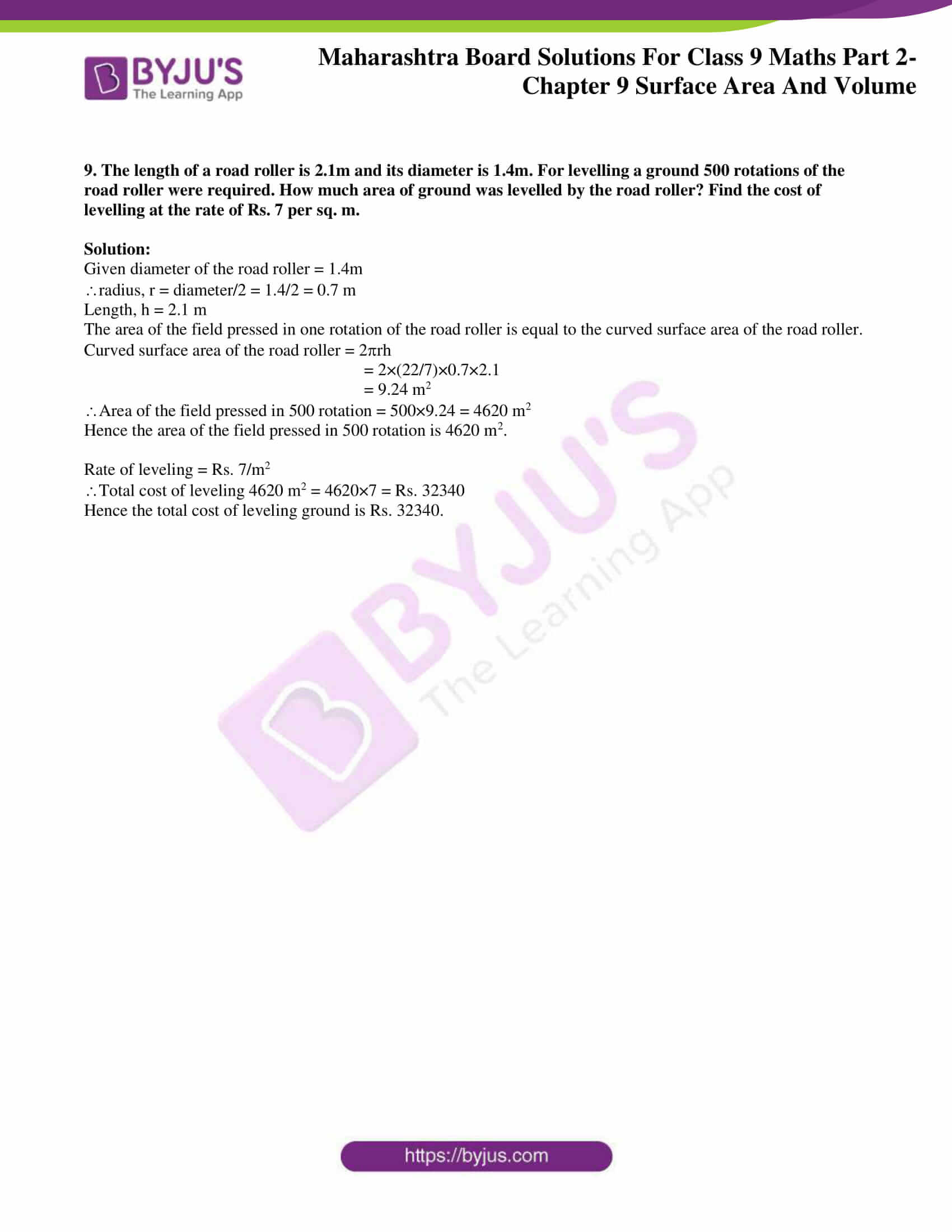### Access answers to Maths MSBSHSE Solutions For Class 9 Part 2 Chapter 9 – Surface Area and Volume

Practice Set 9.1 Page 115

1. Length, breadth and height of a cuboid shape box of medicine is 20cm, 12 cm and 10 cm respectively. Find the surface area of vertical faces and total surface area of this box.

Solution:

Given length of the cuboid shape box of medicine, l = 20 cm

Height, h = 10 cm

Surface area of vertical faces = 2(lh +bh)

= 2(20×10+12×10) = 2(200+120) = 2×320 = 640 cm2

Total surface area of a cuboid = 2(lb+bh+lh)

Total surface area of the box = 2(20×12+12×10+20×10)

= 2 (240+120+200) = 2×560 = 1120 cm2

Hence the surface area of vertical faces and total surface area of the box are 640 cm2 and 1120 cm2.

2. Total surface area of a box of cuboid shape is 500 sq. unit. Its breadth and height is 6 unit and 5 unit respectively. What is the length of that box ?

Solution:

Given Total surface area of the cuboid box = 500

Breadth of the box, b = 6

Height of the box, h = 5

Total surface area of the box = 2(lb+bh+lh)

500 = 2(l×6+6×5+l×5)

500 = 2(6l+30+5l)

500 = 2(11l+30)

500 = 22l+60

22l = 500-60 = 440

l = 440/22 = 20

Hence the length of the box is 20 units.

3. Side of a cube is 4.5 cm. Find the surface area of all vertical faces and total surface area of the cube.

Solution:

Given side of the cube, l = 4.5 cm

Surface area of all vertical faces of the cube = 4l2

= 4×4.52

= 4×20.25

= 81 cm2

Total surface area of the cube = 6l2

= 6×4.52 = 6×20.25

= 121.5 cm2

Hence, the surface area of all vertical faces of the cube and Total surface area of the cube are 81 cm2 and 121.5 cm2 respectively.

4. Total surface area of a cube is 5400 sq. cm. Find the surface area of all vertical faces of the cube.

Solution:

Given total surface area of the cube, = 5400 cm2

Total surface area of the cube = 6l2

6l2 = 5400

l2 = 5400/6 = 900

l = √900 = 30

Surface area of all vertical faces of the cube = 4l2

Surface area of all vertical faces of the cube = 4×302

= 4×900

= 3600 cm2

Hence the Surface area of all vertical faces of the cube is 3600 cm2

5. Volume of a cuboid is 34.50 cubic metre. Breadth and height of the cuboid is 1.5m and 1.15m respectively. Find its length.

Solution:

Given volume of the cuboid, V = 34.50 m3

Breadth of the cuboid, b = 1.5 m

Height of the cuboid, h = 1.15 m

Volume of th cuboid, V = b×h

34.50 = 1.5×1.15

34.50 = 1.725 l

l = 34.50/1.725 = 20 m

Hence the length of the cuboid is 20 m.

6. What will be the volume of a cube having length of edge 7.5 cm ?

Solution:

Given length of the cube, l = 7.5 cm

Volume of the cube, V = l3 = 7.53 = 7.5×7.5×7.5 = 421.875 cm3

Hence, the volume of the cube is 421.875 cm3

7. Radius of base of a cylinder is 20cm and its height is 13cm, find its curved surface area and total surface area. ( = 3.14)

Solution:

Given radius of the cylinder, r = 20 cm

Height of the cylinder, h = 13 cm

Curved surface area of the cylinder = 2rh

= 2×3.14×20×13

= 1632.8 cm2

Total surface area of a cylinder = 2r(r+h )

= 2×3.14×20×(20+13)

= 2×3.14×20×33

= 4144.8 cm2

Hence the curved surface area of the cylinder and total surface area of a cylinder are 1632.8 cm2

and 4144.8 cm2 respectively.

8. Curved surface area of a cylinder is 1980 cm2 and radius of its base is 15cm. Find the height of the cylinder. ( = 22/7 ).

Solution:

Given curved surface area of the cylinder = 1980 cm2

Radius of the cylinder, r = 15 cm

Curved surface area of the cylinder = 2rh

1980 = 2×(22/7)×15×h

h = 1980×7/(2×22×15)

h = 21

Hence the height of the cylinder is 21 cm.

Practice Set 9.2 Page 119

1. Perpendicular height of a cone is 12 cm and its slant height is 13 cm. Find the radius of the base of the cone.

Solution:

Given perpendicular height of a cone, h = 12 cm

Slant height, l = 13 cm

Relation between the slant height, radius and height of a cone is given by the following equation.

l2 = r2+h2

132 = r2+122

r2 = 132-122 = 169-144 = 25

Taking square root on both sides,

r = 5

Hence the radius of the base of the cone is 5 cm.

2. Find the volume of a cone, if its total surface area is 7128 sq.cm and radius of base is 28 cm.

( = 22/7 ).

Solution:

Given total surface area of cone = 7128 cm2

Radius of the cone, r = 28 cm

total surface area of cone = r (l+r)

7128 = (22/7) ×28(l+28)

7128 = 88(l+28)

l+28 = 7128/88

l+28 = 81

l = 81-28 = 53 cm

slant height, l = 53 cm

We know that, l2 = r2+h2

532 = 282+h2

h2 = 532-282

h2 = 532-282

h2 = 2809-784 = 2025

Taking square root on both sides

h = 45

Volume of the cone, V = (1/3)r2h

V = (1/3)×(22/7)×282×45

V = 36960 cm3

Hence the volume of the cube is 36960 cm3.

3. Curved surface area of a cone is 251.2 cm2 and radius of its base is 8cm. Find its slant height and perpendicular height. ( = 3.14 )

Solution:

Given curved surface area of the cone = 251.2 cm2

Radius of the cone, r = 8 cm

Curved surface area of the cone = rl

251.2 = 3.14×8×l

l = 251.2/(3.14×8)

l = 10

slant height, l = 10 cm

We know that, l2 = r2+h2

102 = 82+h2

h2 = 102– 82

h2 = 100-64 = 36

h = 6 cm

perpendicular height = 6 cm

Hence, the slant height and perpendicular height of the cone is 10cm and 6 cm respectively.

4. What will be the cost of making a closed cone of tin sheet having radius of base 6 m and slant height 8 m if the rate of making is Rs.10 per sq.m ?

Solution:

Given radius of the cone, r = 6m

slant height, l = 8 m

To find the cost of making a closed cone, we need to find the total surface area of the cone.

Total surface area of the cone = r(l+r)

= (22/7)×6(8+6)

= (22/7)×6×14

= 22×6×2

= 264 m2

Cost of making the cone per sq.m = Rs.10

Total cost = total surface area × rate of making the cone per sq.m

= 10×264 = Rs. 2640

Hence, the cost of making cone is Rs.2640.

5. Volume of a cone is 6280 cubic cm and base radius of the cone is 30 cm. Find its perpendicular height. ( = 3.14)

Solution:

Given volume of cone, V = 6280 cm3

Base radius of the cone, r = 30 cm

Volume of cone, V = (1/3)r2h

6280 = (1/3)×3.14×302×h

h = (3×6280)/(3.14×900)

h = 6.67

Hence the perpendicular height of the cone is 6.67 approximately.

6. Surface area of a cone is 188.4 sq.cm and its slant height is 10cm. Find its perpendicular height ( = 3.14)

Solution:

Given surface area of a cone = 188.4 cm2

Slant height, l = 10 cm

Surface area of the cone = rl

188.4 = 3.14×r×10

r = 188.4/(3.14×10)

r = 6 cm

We know that, l2 = r2+h2

102 = 62+h2

h2 = 102-62

h2 = 100-36

h2 = 64

Taking square root on both sides

h = 8 cm

Hence, the perpendicular height of the cone is 8 cm.

7. Volume of a cone is 1232 cm3 and its height is 24cm. Find the surface area of the cone. ( = 22/7 )

Solution:

Given volume of the cone, V = 1232 cm3

Height of the cone, h = 24 cm

Volume of the cone, V = (1/3)r2h

1232 = (1/3)×(22/7)×r2×24

r2 = (1232×3×7)/(22×24)

r2 = 49

r = 7

We know that l2 = r2+h2

l2 = 72+242

l2 = 49+576 = 625

l = 25

Surface area of the cone = rl

= (22/7)×7×25 = 550

Hence, the surface area of the cone is 550 cm2.

8. The curved surface area of a cone is 2200 sq.cm and its slant height is 50 cm. Find the total surface area of cone. ( = 22/7)

Solution:

Given curved surface area of a cone = 2200 cm2

Slant height, l = 50 cm

Curved surface area of the cone = rl

2200 = (22/7)×r×50

r = (2200×7)/(22×50)

r = 14 cm

Total surface area of the cone = r(l+r)

= (22/7)×14×(50+14)

= (22/7)×14×64

= 2816 cm2

Hence the total surface area of the cone is 2816 cm2.

9. There are 25 persons in a tent which is conical in shape. Every person needs an area of 4 sq.m. of the ground inside the tent. If height of the tent is 18m, find the volume of the tent.

Solution:

Given height of the tent, h = 18 m

Number of persons in tent = 25

Area needed for one person = 4 m2

Hence, area needed for 25 persons = 25×4 = 100 m2

Here base area of the tent = 100 m2

i.e, r2 = 100

Volume of the conical tent = (1/3)r2h

= (1/3)×100×18 = 600 m3

Hence, the volume of the tent is 600 m3.

10. In a field, dry fodder for the cattle is heaped in a conical shape. The height of the cone is 2.1m. and diameter of base is 7.2 m. Find the volume of the fodder. if it is to be covered by polythene in rainy season then how much minimum polythene sheet is needed ? ( = 22/7 and √17.37 = 4.17)

Solution:

Given the height of the cone, h = 2.1 m

Diameter of the base = 7.2 m

Radius, r = diameter/2 = 7.2/2 = 3.6

Volume of the cone, V = (1/3)r2h

V = (1/3)×(22/7)×3.62×2.1

= 28.51 m3

We know that l2 = r2+h2

l2 = 3.62+2.12

l2 = 12.96+4.41

l2 = 17.37

l = √17.31 = 4.17

To find how much polythene is required to cover the dry fodder, we need to find the curved surface area of the cone.

Curved surface area of the cone = rl

= (22/7)×3.6×4.17

= 47.18 m2

Amount of polythene required = 47.18 m2

Hence, the volume of the fodder is 28.51 m3 and the amount of polythene required is 47.18 m2.

Practice Set 9.3 Page 123

1. Find the surface areas and volumes of spheres of the following radii.

(i) 4 cm (ii) 9 cm (iii) 3.5 cm. ( = 3.14)

Solution:

(i) Given radius of the sphere, r = 4 cm

Surface area of the sphere = 4r2

= 4×3.14×42

= 4×3.14×16

= 200.96 cm2

Volume of the sphere, V = (4/3)r3

= (4/3)×3.14×43

= (4/3)×3.14×64

= 267.946

= 267.95 cm3

Hence, the surface area and volume of the cone are 200.96 cm2 and 267.95 cm3 respectively.

(ii) Given radius of the sphere, r = 9 cm

Surface area of the sphere = 4r2

= 4×3.14×92

= 4×3.14×81

= 1017.36 cm2

Volume of the sphere, V = (4/3)r3

= (4/3)×3.14×93

= (4/3)×3.14×729

= 3052.08 cm3

Hence the surface area and volume of the cone are 1017.36 cm2and 3052.08 cm3 respectively.

(iii) Given radius of the sphere, r = 3.5 cm

Surface area of the sphere = 4r2

= 4×3.14×3.52

= 4×3.14×12.25

= 153.86 cm2

Volume of the sphere, V = (4/3)r3

= (4/3)×3.14×3.53

= (4/3)×3.14×42.875

= 179.50 cm3

Hence the surface area and volume of the cone are 153.86 cm2and 179.50 cm3 respectively.

2. If the radius of a solid hemisphere is 5cm, then find its curved surface area and total surface area. ( = 3.14)

Solution:

Given radius of the solid hemisphere = 5 cm

Curved surface area of the hemisphere = 2r2

= 2×3.14×52

= 157 cm2

Total surface area = 3r2

= 3×3.14×52

= 3×3.14×25

= 235.5 cm2

Hence the Curved surface area and total surface area of the hemisphere are 157 cm2 and 235.5 cm2.

3. If the surface area of a sphere is 2826 cm2 then find its volume. ( = 3.14)

Solution:

Given surface area of a sphere = 2826 cm2

Surface area of the sphere = 4r2

4r2 = 2826 cm2

4×3.14×r2 = 2826

r2 = 2826/(4×3.14)

r2 = 225

Taking square root on both sides

r = 15 cm

Volume of the sphere, V = (4/3)r3

= (4/3)×3.14×153

= (4/3)×3.14×15×15×15

= 14130 cm3

Hence the volume of the sphere is 14130 cm3.

4. Find the surface area of a sphere, if its volume is 38808 cubic cm. ( = 22/7 )

Solution:

Given volume of the sphere = 38808 cm3

Volume of the sphere, V = (4/3)r3

(4/3)r3 = 38808

(4/3)×(22/7)r3 = 38808

r3 = (38808×3×7)/(4×22)

r3 = 9261

Taking cube root on both sides

r = 21

Surface area of the sphere = 4r2

= 4×(22/7)×212

= 4×(22/7)×441

= 5544 cm2

Hence the surface area of the sphere is 5544 cm2.

5. Volume of a hemisphere is 18000 cubic cm. Find its diameter.

Solution:

Given volume of hemisphere = 18000 cm3.

Volume of the hemisphere, V = (2/3)r3

(2/3)r3 = 18000

r3 = 18000×3/2

r3 = 27000

Taking cube root on both sides

r = 30

Diameter = 2×radius = 2×30 = 60 cm

Hence, the diameter of the hemisphere is 60cm.

Problem Set 9 Page 123

1. If diameter of a road roller is 0.9 m and its length is 1.4 m, how much area of a field will be pressed in its 500 rotations ?

Solution:

Given diameter of the road roller = 0.9 m

radius, r = diameter/2 = 0.9/2 = 0.45 m

Length, h = 1.4 m

The area of the field pressed in one rotation of the road roller is equal to the curved surface area of the road roller.

Curved surface area of the road roller = 2rh

= 2×(22/7)×0.45×1.4

= 3.96 m2

Area of the field pressed in 500 rotation = 500×3.96 = 1980 m2

Hence the area of the field pressed in 500 rotation is 1980 m2.

2. To make an open fish tank, a glass sheet of 2 mm gauge is used. The outer length, breadth and height of the tank are 60.4 cm, 40.4 cm and 40.2 cm respectively. How much maximum volume of water will be contained in it ?

Solution:

Given thickness of the glass = 2 mm  = 0.2 cm              [1 cm = 10 mm]

Outer length of the tank = 60.4 cm

Thickness of glass on both sides = 0.2+0.2 = 0.4 cm

Inner length of the tank, l = Outer length of the tank- Thickness of glass on both sides

= 60.4-0.4 = 60 cm

Outer breadth of the tank = 40.4 cm

Inner breadth of the tank, b = Outer breadth of the tank- Thickness of glass on both sides

= 40.4 -0.4 = 40 cm

Inner height of the tank, h = inner height -thickness of glass on bottom side

= 40.2-0.2 = 40 cm               [Tank is open at the top]

Maximum volume of water contained in the tank

= Inner volume of the tank

= l×b×h

= 60×40×40

= 96000 cm3

Hence, the maximum volume of water contained in the tank is 96000 cm3.

3. If the ratio of radius of base and height of a cone is 5:12 and its volume is 314 cubic metre. Find its perpendicular height and slant height ( = 3.14)

Solution:

Given the ratio of the radius and the height of the cone = 5:12

Let x be a common multiple.

Height, h = 12x

Volume of the cone = 314 m3

Volume of the cone = (1/3)r2h

(1/3)r2h = 314

(1/3)(5x)2×12x = 314

(1/3) ×3.14×25x2×12x = 314

x3 = 314×3/(3.14×25×12) = 1

x = 1

= 5×1 = 5 m

Height, h = 12x

= 12×1 = 12 m

We know that l2 = r2+h2

l2 = 52+122 = 25+144 = 169

Taking square root on both sides

l = 13

slant height , l = 13 m

Hence the perpendicular height and slant height of the cone is 12 m and 13 m respectively.

4. Find the radius of a sphere if its volume is 904.32 cubic cm. ( = 3.14)

Solution:

Given volume of a sphere, V = 904.32 cm3

Volume of a sphere, V = (4/3)r3

(4/3)r3 = 904.32

(4/3)×3.14×r3 = 904.32

r3 = (3×904.32)/(4×3.14)

r3 = 216

Taking cube root on both sides

r = 6

Hence, the radius of the sphere is 6 cm.

5. Total surface area of a cube is 864 sq.cm. Find its volume.

Solution:

Given total surface area of a cube = 864 cm2

Total surface area of a cube = 6l2

6l2 = 864

l2 = 864/6 = 144

Taking square root on both sides

l = 12

Volume of a cube = l3

= 123

= 12×12×12

= 1728 cm3

Hence the volume of the cube is 1728 cm3.

6. Find the volume of a sphere, if its surface area is 154 sq.cm.

Solution:

Given surface area of a sphere = 154 cm2

Surface area of a sphere = 4r2

4r2 = 154

4×(22/7)×r2 = 154 [ = 22/7]

r2 = 154×7/(4×22)

r2 = 49/4

Taking square root on both sides

r = 7/2

Volume of the sphere, V = (4/3)r3

= (4/3)×(22/7)×(7/2)3

= 179.67 cm3

Hence, the volume of the sphere is 179.67 cm3.

7. Total surface area of a cone is 616 sq.cm. If the slant height of the cone is three times the radius of its base, find its slant height.

Solution:

Given slant height is three times the radius of the base.

Slant height, l = 3r

Total surface area of the cone = r(r+l)

= r(r+3r)

= r(4r)

= 4r2

Given total surface area of a cone = 616 cm2

4r2 = 616

4×(22/7)×r2 = 616

r2 = 616×7/(4×22)

r2 = 49

Taking square root on both sides,

r = 7 cm

Slant height, l = 3r

= 3×7

= 21 cm

Hence, the slant height of the cone is 21 cm.

8. The inner diameter of a well is 4.20 metre and its depth is 10 metre. Find the inner surface area of the well. Find the cost of plastering it from inside at the rate Rs.52 per sq.m.

Solution:

Given inner diameter = 4.20 m

radius, r = 4.20/2 = 2.10 m

Depth of the well, h = 10 m

Inner surface area of the wall = 2rh

= 2×(22/7)×2.10×10

= 132 m2

Cost of plastering per square metre = Rs. 52

Cost of plastering 132 square metre = 132× 52= 6864

Hence the cost of plastering the well from inside is Rs. 6864.

9. The length of a road roller is 2.1m and its diameter is 1.4m. For levelling a ground 500 rotations of the road roller were required. How much area of ground was levelled by the road roller? Find the cost of levelling at the rate of Rs. 7 per sq. m.

Solution:

Given diameter of the road roller = 1.4m

radius, r = diameter/2 = 1.4/2 = 0.7 m

Length, h = 2.1 m

The area of the field pressed in one rotation of the road roller is equal to the curved surface area of the road roller.

Curved surface area of the road roller = 2rh

= 2×(22/7)×0.7×2.1

= 9.24 m2

Area of the field pressed in 500 rotation = 500×9.24 = 4620 m2

Hence, the area of the field pressed in 500 rotation is 4620 m2.

Rate of leveling = Rs. 7/m2

Total cost of leveling 4620 m2 = 4620×7 = Rs. 32340

Hence, the total cost of leveling ground is Rs. 32340.

This chapter explains the volume of a sphere and hemisphere. It also describes how to find the curved surface area and total surface area of sphere, cone and hemisphere. Sign in to BYJU’S to get more ideas on Surface Area and Volume.

## Frequently Asked Questions on Maharashtra State Board Solutions for Class 9 Solutions Maths Part 2 Chapter 9 Surface Area and Volume

### Are these Maharashtra State Board Solutions for Class 9 Maths Part 2 Chapter 9 Surface Area and Volume helpful to prepare for the exams?

Yes, these solutions are very helpful. Students are recommended practising these solutions after revising the subjects, for they set the basis for the questions that could get asked often in the board exams. These solutions also act as a perfect guide for Class 9 students during their board exam preparation because it is created by qualified subject teachers according to the latest class 9 Maths Part 2 Syllabus.